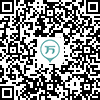143:002020年成人高考（专升本）《大学语文》真题
1～20小题，每小题2分，共40分。在每小题给出的四个选项中，只有一项是符合题目要求的，把所选项前的字母填在题后的括号内。
1
(单项选择题)

• A.

《季氏将伐颛臾》

• B.

《寡人之于国也》

• C.

《道德经》

• D.

《礼记•大同》

• A
• B
• C
• D

2
(单项选择题)

• A.

《冯谖客孟尝君》

• B.

《陈情表》

• C.

《灯下漫笔》

• D.

《选择与安排》

• A
• B
• C
• D

3
(单项选择题)

• A.

《发现》

• B.

《炉中煤》

• C.

《我爱这土地》

• D.

《再别康桥》

• A
• B
• C
• D

4
(单项选择题)

• A.

福克纳

• B.

卡夫卡

• C.

莫泊桑

• D.

狄更斯

• A
• B
• C
• D

5
(单项选择题)

• A.

沈从文

• B.

巴金

• C.

曹禺

• D.

老舍

• A
• B
• C
• D

6
(单项选择题)

• A.

主张“有补于世”

• B.

北宋著名政治家

• C.

著有《资治通鉴》

• D.

唐宋八大家之一

• A
• B
• C
• D

7
(单项选择题)

• A.

朱自清

• B.

郭沫若

• C.

茅盾

• D.

徐志摩

• A
• B
• C
• D

8
(单项选择题)

• A.

赋经常交互使用散文句与对偶句

• B.

赋行文比较灵活，不讲究押韵

• C.

赋多采用主客问答的方式，以表达主人的意见为主

• D.

赋多用抑客伸主的形式进行表述

• A
• B
• C
• D

9
(单项选择题)

• A.

“王好战，请以战喻”，侧面指出了梁惠王好战致使民不聊生

• B.

“养生丧死无憾，王道之始也”，说明人民安居乐业的重要性

• C.

“寡人之民不加多”，是因为梁惠王对治理国家不用心不用力

• D.

“是何异于刺人而杀之”，指出了统治阶级对人民的隐性剥削

• A
• B
• C
• D

10
(单项选择题)

• A.

孔子认为颛臾是东蒙之主不能伐

• B.

孔子认为颛臾在邦域之内不必伐

• C.

孔子认为颛臾是季氏子孙的忧患

• D.

孔子认为颛臾是社稷之臣不应伐

• A
• B
• C
• D

11
(单项选择题)

• A.

“对酒当歌，人生几何”表达了诗人对人生短暂的感慨

• B.

“绕树三匝，何枝可依”表达了诗人不愿再漂泊的心情

• C.

“青青子衿，悠悠我心”抒发了诗人求贤若渴的心情

• D.

“周公吐哺，天下归心”展现了诗人礼贤下士的胸怀

• A
• B
• C
• D

12
(单项选择题)

• A.

情绪低迷，徘徊不已，又自然、深切、动人

• B.

大胆新奇，独具一格，让人感到陌生

• C.

充满音乐性，给人“大珠小珠落玉盘”的感受

• D.

营造出黄昏细雨的诗情，烘托诗人内心最终浮现的点滴喜悦

• A
• B
• C
• D

13
(单项选择题)

• A.

贾母责骂贾政并要人备车回南京

• B.

王夫人的哭诉让李纨不禁悲痛

• C.

薛宝钗因说到薛蟠而对宝玉心生不满

• D.

林黛玉看望宝玉时因怕被取笑而匆匆离去

• A
• B
• C
• D

14
(单项选择题)

• A.

淇则有岸，隰则有泮

• B.

女也不爽，士贰其行

• C.

桑之未落，其叶沃若

• D.

于嗟鸠兮，无食桑葚

• A
• B
• C
• D

15
(单项选择题)

“忽如一夜春风来，千树万树梨花开”使用的修辞方法是（　）。

• A.

比喻

• B.

拟人

• C.

用典

• D.

对偶

• A
• B
• C
• D

16
(单项选择题)

• A.

憔悴损，如今有谁【堪】摘

国不【堪】贰，君将若之何

• B.

春蚕到死丝【方】尽，蜡炬成灰泪始干

昨日里胥【方】到门，手持尺牒榜乡村

• C.

举酒【属】客，诵明月之诗，歌窈窕之章

使人【属】孟尝君，愿寄食门下

• D.

不违农时，谷不可胜【食】也

左右以君贱之，【食】以草具

• A
• B
• C
• D

17
(单项选择题)

• A.

问君何能【尔】，心远地自偏

尔：同“迩”

• B.

【何以】解忧？唯有杜康

何以：以何

• C.

井蛙不可【以】语于海者

以：与

• D.

朱门沉沉【按】歌舞，厩马肥死弓断弦

按：歌舞时打拍子

• A
• B
• C
• D

18
(单项选择题)

• A.

是社稷之臣也，何以伐【为】

• B.

臣闻吏议逐客，窃以【为】过矣

• C.

广为陇西都尉，徙【为】骑郎将

• D.

而身死国灭，【为】天下笑

• A
• B
• C
• D

19
(单项选择题)

• A.

寻蒙国恩，【除臣洗马】，猥以微贱

除臣洗马：开除臣子命令他为别人洗马

• B.

将出城，抽矢【射佛寺浮图】，矢著其上砖半箭

射佛寺浮图：射中佛寺上面的浮雕图画

• C.

乘纤离之马，【建翠凤之旗】

翠凤之旗：绣着绿凤凰的旗帜

• D.

昔者仲尼【与于蜡宾】，事毕，出游于观之上

与于蜡宾：参加到陪祭者的行列里

• A
• B
• C
• D

20
(单项选择题)

• A.

其培之也，若不过焉则不及

• B.

今皆解鞍以示不走，用坚其意

• C.

各亲其亲，各子其子，货力为己

• D.

今乃弃黔首以资敌国，却宾客以业诸侯

• A
• B
• C
• D